﻿ 【项目实践】商业计算怎样才能保证精度不丢失_其他_开心洋葱网
• 欢迎访问开心洋葱网站，在线教程，推荐使用最新版火狐浏览器和Chrome浏览器访问本网站，欢迎加入开心洋葱` QQ群`
• 为方便开心洋葱网用户，开心洋葱官网已经开启复制功能！
• 欢迎访问开心洋葱网站，手机也能访问哦~欢迎加入开心洋葱多维思维学习平台` QQ群`
• 如果您觉得本站非常有看点，那么赶紧使用Ctrl+D 收藏开心洋葱吧~~~~~~~~~~~~~！
• 由于近期流量激增，小站的ECS没能经的起亲们的访问，本站依然没有盈利，如果各位看如果觉着文字不错，还请看官给小站打个赏~~~~~~~~~~~~~！

# 【项目实践】商业计算怎样才能保证精度不丢失

1480次浏览# 前言

``````double money = 1.0 - 0.9;
``````

# 解决方案

## Decimal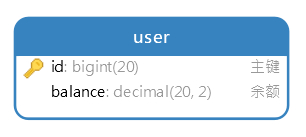``````BigDecimal d1 = new BigDecimal(0.1); // BigDecimal(double val)
BigDecimal d2 = new BigDecimal("0.1"); // BigDecimal(String val)
BigDecimal d3 = BigDecimal.valueOf(0.1); // static BigDecimal valueOf(double val)
``````

``````d1: 0.1000000000000000055511151231257827021181583404541015625
d2: 0.1
d3: 0.1
``````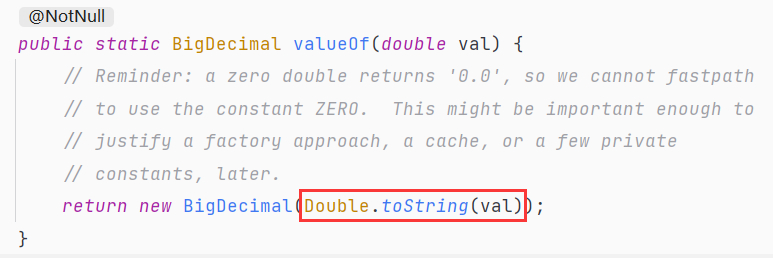``````BigDecimal add(BigDecimal); // 加
BigDecimal subtract(BigDecimal); // 减
BigDecimal multiply(BigDecimal); // 乘
BigDecimal divide(BigDecimal); // 除
``````

`BigDecimal` 是不可变对象，意思就是这些操作都不会改变原有对象的值，方法执行完毕只会返回一个新的对象。若要运算后更新原有值，只能重新赋值：

``````d1 = d1.subtract(d2);
``````

``````BigDecimal d1 = new BigDecimal("1.0");
BigDecimal d2 = new BigDecimal("0.9");
System.out.println(d1.subtract(d2));
``````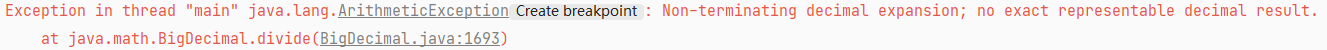``````BigDecimal divide(BigDecimal divisor, int scale, int roundingMode)
``````

`scale` 参数表示运算后保留几位小数，`roundingMode` 参数表示计算小数的方式。

``````BigDecimal d1 = new BigDecimal("1.0");
BigDecimal d2 = new BigDecimal("3");
System.out.println(d1.divide(d2, 2, RoundingMode.DOWN)); // 小数精度为2，多余小数直接舍去。输出结果为0.33
``````

`RoundingMode` 枚举能够方便地指定小数运算方式，除了直接舍去，还有四舍五入、向上取整等多种方式，根据具体业务需求指定即可。

``````BigDecimal d1 = new BigDecimal("0.33");
BigDecimal d2 = new BigDecimal("0.3300");
System.out.println(d1.equals(d2)); // false
``````

``````BigDecimal d1 = new BigDecimal("0.33");
BigDecimal d2 = new BigDecimal("0.3300");
System.out.println(d1.compareTo(d2) == 0); // true
``````

`d1` 大于 `d2`，返回 `1`

`d1` 小于 `d2`，返回 `-1`

`BigDecimal` 的用法就介绍到这，我们接下来看第二种解决方案。

## 定长整数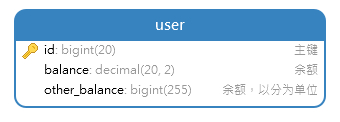``````long d1 = 10000L; // 100元
d1 += 500L; // 加五元
d1 -= 500L; // 减五元
``````

``````long d1 = 2366L; // 23.66元
double result = d1 * 0.8; // 打八折，运算后结果为1892.8
d1 = (long)result; // 转换为整数，舍去所有小数，值为1892。即18.92元
``````

``````long d1 = 2366L; // 23.66元
double result = d1 * 0.8; // 运算后结果为1892.8
d1 = (long)result; // 强转舍去所有小数，值为1892
d1 = (long)Math.ceil(result); // 向上取整，值为1893
d1 = (long)Math.round(result); // 四舍五入，值为1893
...
``````

``````long d1 = 2366L;
long result = d1 / 3; // 正确的值本应该为788.6666666666666，舍去所有小数，最终值为788
``````

``````long d1 = 2366L;
double result = d1 / 3.0; // 注意，这里除以不是 3，而是 3.0 浮点数
d1 = (long)Math.round(result); // 四射勿入，最终值为789，即7.89元
``````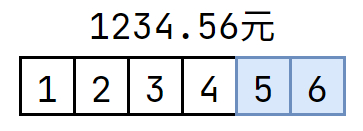# 收尾

• Decimal 类型
• 定长整数